# COUNTIFS Function

Counts the number of cells that meet a single or multiple criteria

## What is the COUNTIFS Function?

The COUNTIFS function is categorized under Excel Statistical functions. COUNTIFS will count the number of cells that meet a single criterion or multiple criteria in the same or different ranges.

The difference between COUNTIF and COUNTIFS is that COUNTIF is designed for counting cells with a single condition in one range, whereas COUNTIFS can evaluate different criteria in the same or different ranges.

When doing financial analysis, COUNTIF helps in doing a quick analysis. For example, we are given a list of tasks to be completed by a department, and the dates and priority of each task. In such a scenario, we can prepare a table showing the date, count of each task, and their priority using the COUNTIFS function.

### Formula

=COUNTIFS(criteria_range1, criteria1, [criteria_range2, criteria2]…)

The COUNTIFS function uses the following arguments:

1. Criteria_range1 (required argument) – This is the first range that would be evaluated with the associated criteria.
2. Criteria1 (required argument) – The conditions to be tested against the values. The criteria can be in the form of a number, expression, cell reference, or text that define which cells shall be counted. For example, criteria can be expressed as 2, “>2,” A4, “Mangoes,” or “32.”
3. Criteria_range2, criteria2, … (optional argument) – Additional ranges and their associated criteria. The function allows up to 127 range/criteria pairs.

The given criteria can be any of the following:

1. It is a numeric value. It can be an integer, decimal, date, time, or logical value.
2. A text string. It may include wildcards. Wildcards can be a ? (question mark) or an * (asterisk). A ? matches any single character, whereas, * matches any sequence of characters. If we wish to actually find the ? (question mark) or * (asterisk) character, we need to type the ~ symbol before this character in our search.

### How to use the COUNTIFS Function?

As a worksheet function, the COUNTIFS function can be entered as part of a formula in a cell of a worksheet.

To understand the uses of the function, let’s consider a few examples:

#### Example 1

Let’s assume we are given the following data: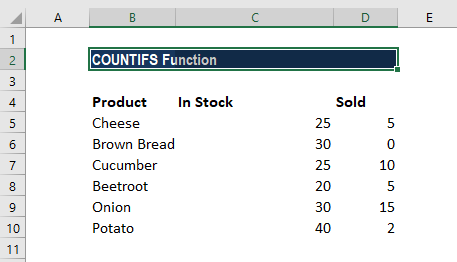We want to get a count of items that are in stock (value in column C is greater than 0) but remain unsold (value is column D is equal to 0).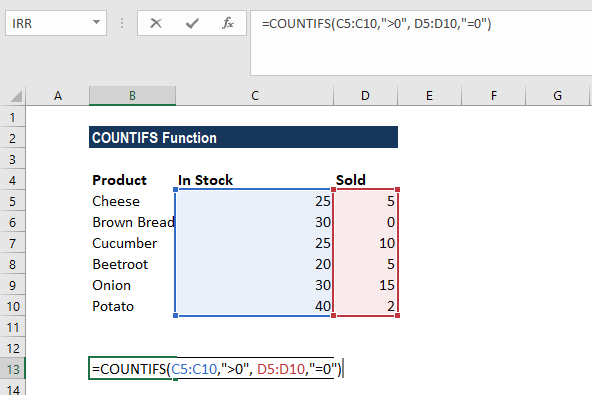Here, as we can see, it’s only Brown Bread where no stock is sold. The result we get is: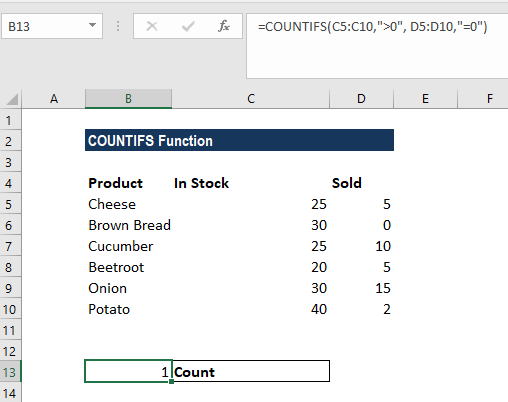#### Example 2

Let’s create count by month. For that, we need to use two functions – the COUNTIFS function and the EDATE function with two criteria.

Suppose we are given the following data: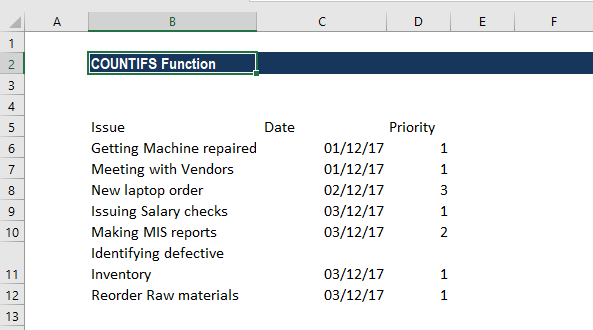The list of issues is in Column B. Each issue includes a date (column C) and priority (column D).

Starting in cell B15, we prepared a summary table that shows a total count per day and a total count per day per priority.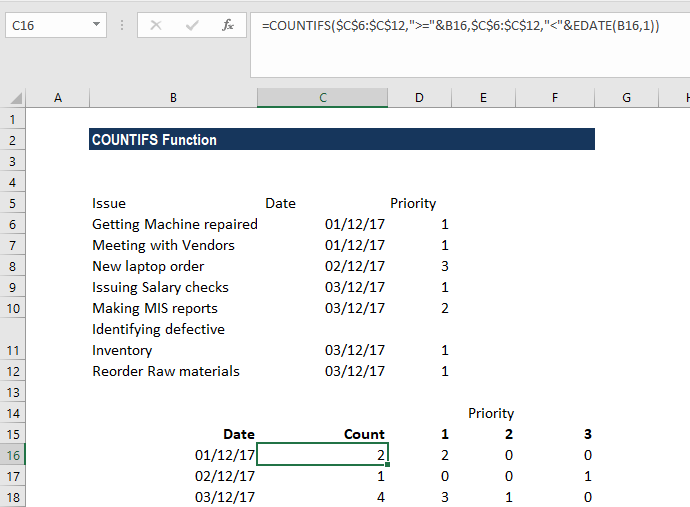The first column of the summary table is a date. With the actual dates in column C, we can easily construct the criteria we need using the date itself and a second date created with the EDATE function.

The formula used here is: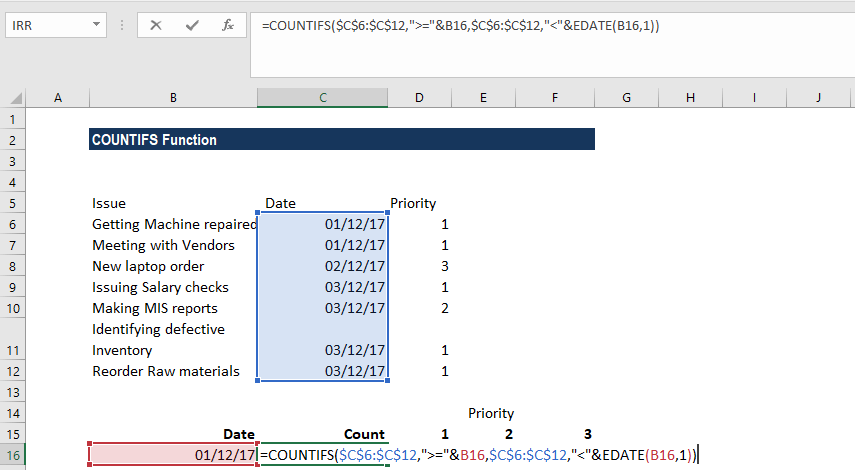The formula generates the correct count for each date. For priorities, we used the formula below: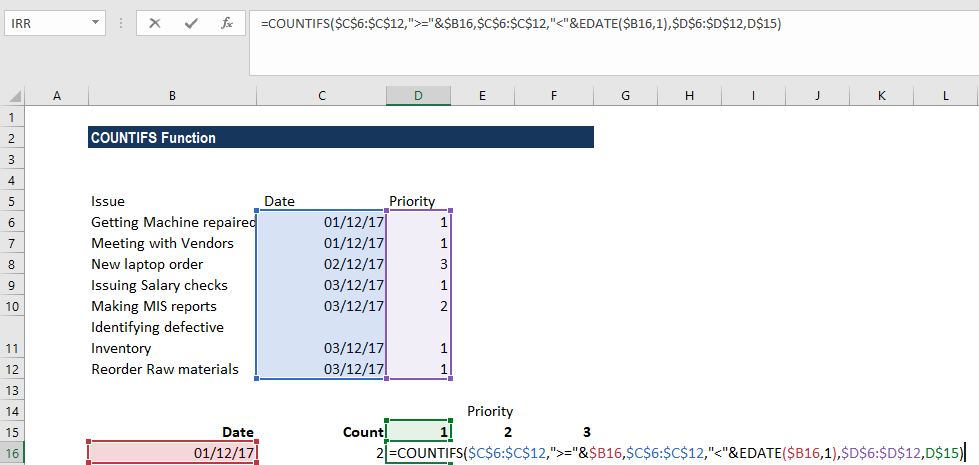The formula returns a count by date but broken down by priority, which is picked up directly from row 6.

The end result is the number of tasks to be done in terms of priority-wise: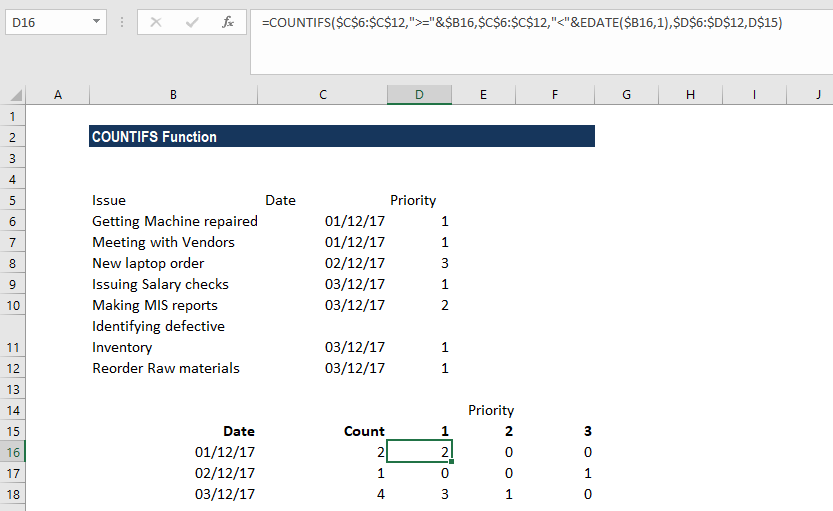### Some notes about the COUNTIFS Function:

1. COUNTIFS is available from MS Excel 2000.
2. #VALUE! error – Occurs when:
• The given criteria_range arrays are not equal in length.
• The supplied criteria arguments are text strings that are greater than 255 characters long.
3. Each additional range should include the same number of rows and columns as the criteria_range1 argument, although it is necessary that they aren’t adjacent to each other.

Thanks for reading CFI’s guide to important Excel functions! By taking the time to learn and master these functions, you’ll significantly speed up your financial analysis. To learn more, check out these additional CFI resources:

• Excel Functions for Finance